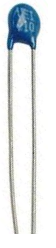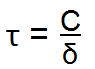﻿ Thermal Time Constant Calculator ﻿# Thermal Time Constant Calculatornan s

The thermal time constant calculator calculates the time in seconds it takes for a thermistor to change 63.2% of the total difference between its initial and final body temperature when subjected to a step function change in temperature, under zero power conditions.

So basically the thermal time constant is the amount of time it takes for a thermistor to register a 63.2% change in temperature when no longer exposed to any power.

A common method of measuring thermal time constant of a thermistor is placing the device at room temperature. Power (or heat) is then applied to raise the thermistor's body temperature, so that is great deal above the ambient air temperature. Once the thermistor's body temperature is raised and sustained at this elevated temperature, power is then removed. At this instantaneous point, a timer should be set off. This is the point where time measure should begin. Once the body has lowered to the temperature which is 63.2% of the temperature difference between the elevated temperature and the ambient, time should be stopped. This time period represents one thermal time constant.

The factors which affect the thermal time constant of a thermistor is its physical size, thermal conductivity, and its thermal mass. Generally, the larger the physical size of the thermistor, the longer the themrmal response time will be. This is because a larger sized thermistor is bigger; therefore, it takes longer to discharge its heat. Thermal mass has the same direct relationship. The larger the mass, the longer the time constant, for the same reason.

To use this calculator, a user enters in the thermal capacity, C, of the thermistor and its dissipation coefficient, and then the result is automatically computed. The resultant value computed is in unit seconds (s).

Related Resources

﻿